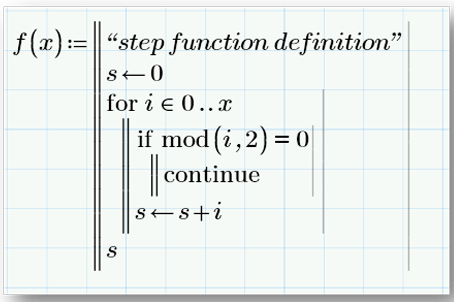<img src="http://www.66infra-strat.com/79795.png?trk_user=79795&amp;trk_tit=jsdisabled&amp;trk_ref=jsdisabled&amp;trk_loc=jsdisabled" height="0px" width="0px" style="display:none;">

#### Concurrent Engineering BlogIn every field of engineering, calculations are essential. Sometimes these calculations are simple, and performed using a notepad and engineering calculator or, more likely, a spreadsheet program like Excel. But, engineering calculations, much like life, are not always simple. Calculations have to take into account more than just the technical aspects of the task in hand and also consider other aspects such as efficiency and cost. Lets take, for example, the complex calculations needed to design an electric motor or transformer. You need to consider the type of lamination iron to be used, the size of the core, type of insulation, and chose between aluminium or copper conductors. During the calculation process, you have to tie all these elements together to optimise the design, taking into account efficiency, cooling, and cost.

## Spreadsheet solutions for engineering calculations

Spreadsheets are a common solution used to handle engineering calculations. They can work well, but only to a point. There are several negatives aspects to consider. Some of the difficulties include:

• Spreadsheet Design: Where interrelated variables exist, the design of the spreadsheet can be difficult, especially if the calculation is limited to one worksheet, inhibiting a simple, readable layout

• Verification of formulae: It is difficult to verify spreadsheet formulae because they are hidden in cells, and interpreting functions can take a bit of time, especially those that are complex.

• Units: Conversion of units can be time consuming and care needs to be taken to ensure consistency or the results will be incorrect

• Annotation: Although text descriptions can be added to explain the functions and formulae in cells, they can be clumsy and confusing to understand.

• Not User Friendly: When spreadsheets are used to represent complex formulae, they are often difficult to read. Setting up a complex calculation is tedious; especially where specialised cell formatting is used to highlight errors.

## The Real Alternative: Engineering Calculation Software

Engineering calculation software is designed so that it can be intuitively understood by engineers familiar with manual calculation methods. It presents results in a readable and easily interpreted form. Formulae are written just as they would be in textbooks. Using an engineering calculations software package such as Mathcad Prime 3.0, enables engineers are able to really transform their engineering calculation processes:

• Clarity: Whereas in spreadsheets like Excel a great deal of information is hidden within the cells, formulae entered into Mathcad are obvious and clear. They are presented as they would appear on paper or on the blackboard.

• Calculation Layout: Mathcad uses blocks called Solve blocks that can be moved around freely without affecting dependencies or other parts of the calculation. If you want to change the order of the blocks you can do so with a click and drag of the mouse.

• Annotation: Annotation is easy and can be written so that the formula is part of the annotation, giving a clear and readable result.

• Consistent Units: Simply tell Mathcad what units you are using and it checks the calculation to make sure that the use of units is consistent and correct.

• Import and Export to Excel: Mathcad can import and export information to Excel.

• Complex formulae: Complicated formulae are much easier to write and visualise in Mathcad than in a spreadsheet application.

• Sharing with CAD: Mathcad worksheets can integrate with other PTC products such as PTC Creo Parametric and Windchill.

Want to find out more about the benefits of engineering calculations software? Take a look at the Mathcad Prime 3.0 Migration Guide: